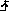# Triggered To Workspace

Write input sample to MATLAB workspace when triggered

## Library

Sinks

`dspsnks4`

•## Description

The Triggered To Workspace block creates a matrix or array variable in the MATLAB® workspace, where it stores the acquired inputs at the end of a simulation. The block overwrites an existing variable with the same name.

When you set the Save 2-D signals as parameter to `2-D array (concatenate along first dimension`, the block saves an M-by-N input as a P-by-N matrix, where P is the Maximum number of rows parameter. When the simulation progresses long enough for the block to acquire more than P samples, the block stores only the most recent P samples. The Decimation factor, D, allows you to store only every Dth input matrix.

When you set the Save 2-D signals as parameter to `3-D array (concatenate along third dimension`, the block saves an M-by-N input as a three-dimensional array in which each M-by-N page represents a single sample from each of the M*N channels (the most recent input matrix occupies the last page). The maximum size of this variable is limited to M-by-N-by-P, where P is the Maximum number of rows parameter. When the simulation progresses long enough for the block to acquire more than P inputs, it stores only the last P inputs. The Decimation factor, D, allows you to store only every Dth input matrix.

The block acquires and buffers a single frame from input 1 whenever it is triggered by the control signal at input 2 (). At all other times, the block ignores input 1. You specify the triggering event at input 2 in the Trigger type pop-up menu:

• `Rising edge` triggers execution of the block when the trigger input rises from a negative value to zero or a positive value, or from zero to a positive value.

• `Falling edge` triggers execution of the block when the trigger input falls from a positive value to zero or a negative value, or from zero to a negative value.

• `Either edge` triggers execution of the block when either a rising or falling edge (as described above) occurs.

To save a record of the sample time corresponding to each sample value, open the Configuration Parameters dialog box. In the Select pane, click and select the Time check box.

The nontriggered version of this block is the To Workspace (Simulink) block.

## Parameters

Trigger type

The type of event that triggers the block's execution.

Variable name

The name of the workspace variable in which to store the data.

Maximum number of rows

The maximum number of rows (one row per time step) to be saved, P.

Decimation

The decimation factor, D.

Save 2-D signals as

Specify whether the block saves 2-D signals as a 2-D or 3-D array in the MATLAB workspace:

• ```2-D array (concatenate along first dimension)``` — When you select this option, the block vertically concatenates each M-by-N matrix input with the previous input to produce a 2-D output array.

• ```3-D array (concatenate along third dimension)``` — When you select this option, the block saves an M-by-N input signal as a 3-D array. The maximum size of this 3-D array is limited to M-by-N-by-P, where P is the Maximum number of rows parameter. When the simulation progresses long enough for the block to acquire more than P inputs, the block stores only the last P inputs. The Decimation factor, D, allows you to store only every Dth input matrix.

Log fixed-point data as a fi object

Select to log fixed-point data to the MATLAB workspace as a Fixed-Point Designer™ `fi` object. Otherwise, fixed-point data is logged to the workspace as `double`.

## Supported Data Types

PortSupported Data Types

Input

• Any data type supported by the To Workspace (Simulink) block

Trigger

• Any data type supported by the Trigger (Simulink) block

 Signal From Workspace DSP System Toolbox To Workspace (Simulink) Simulink Triggered Signal From Workspace DSP System Toolbox

## Extended Capabilities

### Fixed-Point ConversionDesign and simulate fixed-point systems using Fixed-Point Designer™.

Introduced before R2006a

## SupportGet trial now